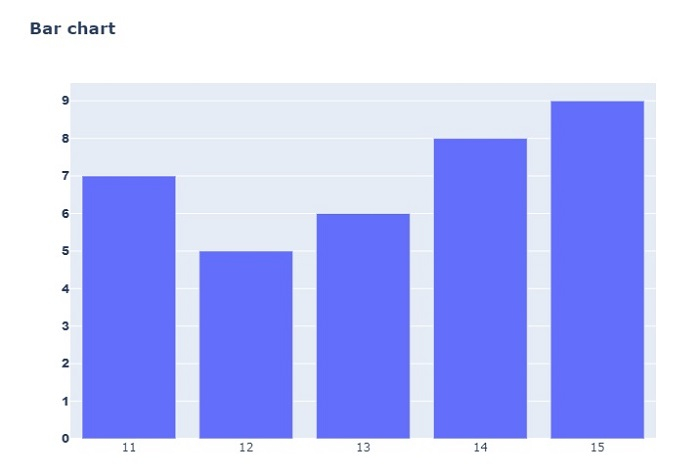# How to set the font style to Bold in Python Plotly?

Plotly is an open-source Python library for creating charts. You can use its features to customize the fonts in various formats. In this tutorial, we will show how you can set the font style to Bold in Python Plotly.

• Here, we will use the plotly.graph_objects module to generate figures. It contains a lot of methods to customize the charts and render them into HTML format.

• Then we will use the update_layout() method to set the title as bold format with <b> tag.

Follow the steps given below to set the font style to bold in Plotly.

Step 1

Import the plotly.graphs_objs module and alias as go.

import plotly.graphs_objs as go


Step 2

Create a dataframe using the following coordinates −

data = {
'species':[12,13,11,14,15],
'age':[5,6,7,8,9]
}
df = pd.DataFrame(data)


Step 3

Create a Bar chart with the following X and Y axis values

fig = go.Figure(go.Bar(
x=df['species'],
y=df['age'])
)


Step 4

Use the update_layout() method to set the title of the bar chart in bold.

fig.update_layout(title='<b>Bar chart</b>')


Step 5

Set the Y-axis label with bold font family as defined below −

fig.update_yaxes(tickfont_family="Arial Black")


Finally, display the chart using fig.show().

## Example

The complete code to set the font style to bold is as follows −

import plotly.graph_objects as go
import pandas as pd
data = {
'species':[12,13,11,14,15],
'age':[5,6,7,8,9]
}
df = pd.DataFrame(data)

fig = go.Figure(go.Bar(
x=df['species'],
y=df['age'])
)
fig.update_layout(title='Bar chart')
fig.update_yaxes(tickfont_family="Arial Black")

fig.show()


## Output

On execution, it will show the following output on the browser −Notice that the Y-axis labels in the chart are set in bold.